Balbharti Maharashtra State Board Bookkeeping and Accountancy 11th Solutions Chapter 7 Depreciation Textbook Exercise Questions and Answers.

## Maharashtra State Board Bookkeeping and Accountancy 11th Solutions Chapter 7 Depreciation

1. Answer in One Sentence only.

Question 1.
What is depreciation?
Depreciation is a gradual, continuous, and permanent decline or decrease in the value of a fixed asset due to its use, wear and tear or any other similar reason.

Question 2.
Why depreciation is charged?
Depreciation on fixed assets is charged to ascertain the correct profit or loss on its sale, to show assets at the correct value in the Balance sheet, and to provide for its replacement.Question 3.
What is a ‘Scrap Value’ of an asset?
The total amount whatsoever received by selling used or obsolete assets or their spare parts is called residual.

Question 4.
Why depreciation is charged even in the year of loss?
Fixed assets are used even in the year of loss and the use of fixed assets reduces its value and hence depreciation is charged even in the year of loss.

Question 5.
Which account is credited when depreciation is charged?
The concerned fixed asset account is credited when depreciation is charged.

Question 6.
Where is the profit or loss on sale of the asset is transferred?
The profit or loss on the sale of assets is transferred to the profit and loss account.

Question 7.
To which account balance of Depreciation A/c is transferred?
The balance of Depreciation A/c is transferred to profit and loss A/c at the end of the year.

Question 8.
What is the formula to calculate depreciation by the Straight Line Method?
Depreciation per annum = $$\frac{Cost of Fixed Asset (-) Scrap value}{Estimated life of Fixed Asset}$$Question 9.
What is Fixed Instalment Method?
A method of charging depreciation in which depreciation is charged on fixed assets at a fixed percentage of its original cost is called the fixed installment method.

Question 10.
Which account is debited when expenses are paid on the installation of the Machinery?
The machinery account is debited when expenses are paid for the installation of machinery.

2. Write the word/term/phrase which can substitute each of the following statements:

Question 1.
A continuous, gradual, and permanent reduction in the value of the fixed assets.
Depreciation

Question 2.
The expenditure incurred for purchase, installation charges, etc. of an asset.
Cost of Asset

Question 3.
The amount that a fixed asset is expected to realize at its disposal.
Scrap Value

Question 4.
The period for which the asset remains in working condition.
The life period of assetQuestion 5.
The method of depreciation in which the total depreciation is equally spread over the life of the asset.
Fixed Instalment Method

Question 6.
The method of depreciation in which the rate of depreciation is fixed but the amount of depreciation reduces every year.
Reducing Balance Method

Question 7.
The type of asset on which depreciation is charged.
Fixed Asset

Question 8.
Expenses incurred for fixation of the new asset to bring it in working condition.
Installation Charges

Question 9.
Excess of the Selling price of a fixed asset over its Written Down Value.
Profit on Sale of Asset

Question 10.
Method of depreciation that cannot reach zero value.
Diminishing Balance Method

3. Select the most appropriate answers from the alternatives given below and rewrite the sentence.

Question 1.
Decrease in the value of fixed assets is known as _____________
(a) Depreciation
(b) Appreciation
(c) Combination
(d) None of these
(a) DepreciationQuestion 2.
Depreciation is charged only on _____________ assets.
(a) Fixed
(b) Current
(c) Non-performing
(d) Fictitious
(a) Fixed

Question 3.
The amount spent on installation of new machinery is a _____________ expenditure.
(a) Revenue
(b) Capital
(c) Deferred Revenue
(d) Income
(b) Capital

Question 4.
The amount that a fixed asset is expected to realise on its disposal is known as _____________
(a) Book value
(b) Scrap value
(c) Market value
(d) Original value
(b) Scrap value

Question 5.
The amount of depreciation reduces year after year under _____________
(a) Fixed Instalment Method
(b) Written Down Value Method
(c) Depreciation Fund Method
(d) Revaluation Method
(b) Written Down Value Method

Question 6.
The amount of depreciation remains constant every year under _____________
(a) Straight Line Method
(b) Diminishing Balance Method
(c) Revaluation Method
(d) Insurance Policy Method
(a) Straight Line MethodQuestion 7.
The balance of depreciation account is transferred to _____________
(a) Manufacturing A/c
(c) Profit & Loss A/c
(d) Balance sheet
(c) Profit and Loss A/c

4. State whether the following statements are True or False with reasons.

Question 1.
Depreciation is charged on fixed assets.
This statement is True.
Fixed assets working life is longer. The working life of all fixed assets decreases with the passage of time. The value of assets decreases every year so a reduction in the value of fixed assets due to its wear and tear depreciation is charged on fixed assets.

Question 2.
Depreciation increases the value of the asset.
This statement is False.
Depreciation reduced the value of the fixed assets. The working life of all fixed assets decreases with the passage of time and its wear and tear.

Question 3.
Balance of the depreciation account is transferred to Profit and Loss A/c.
This statement is True.
Depreciation is charged to profit and Loss A/c as it is an element of Cost. It is also essential to arrive at true value of the asset and also net profit or Loss during a particular accounting period. Even if an asset is not in use, its value is reduced due to the passage of time. Depreciation is Cost/Loss to the business. It is a noncash expenditure.

Question 4.
The Profit or Loss on the sale of the asset is ascertained only after charging depreciation.
This statement is True.
Cost on date of sale can be ascertained only after deducting depreciation from date of purchase till the date of sale after that it is possible to compare between cost on the date of sale and selling price to ascertain profit or loss on sale of the machine.

Question 5.
Wages paid for the installation of Machinery are debited to Wages A/c.
This statement is False.
Wages paid on the installation of machinery are debited to the machinery account as they are the capital nature of expenditures.

Question 6.
It is not necessary to depreciate an asset if it is not in use.
This statement is False.
The working life of fixed assets decreases with passes of time. The value of these assets decreases every year as new technology introduced in the market old becomes outdated so it is necessary to depreciate an asset even it is not in use.Question 7.
Depreciation is charged on Current Assets only.
This statement is False.
Depreciation is charged only on fixed assets and not on current assets working life of fixed assets is longer and it decreases with passes of time. The value of fixed assets decreases every year so depreciation is charged on fixed assets.

Question 8.
Depreciation need not be charged when a business is making a loss.
This statement is False.
Depreciation is charged whether a business is making losses or profits. Depreciation is the non-cash expenditure of the business like all other expenses are charged in the same way depreciation is charge even business is making losses.

5. Complete the following sentences.

Question 1.
Depreciation is charged on _____________ asset.
Fixed

Question 2.
Wages paid for Installation/fixation of Machinery is debited to _____________ account.
Machinery

Question 3.
Under _____________ system, the amount of depreciation changes every year.
Diminishing Balance

Question 4.
Depreciation = $$\frac{Cost of Asset Less …………}{Estimated Working Life of Asset}$$
Scrap value

Question 5.
Gradual and permanent decrease in the value of asset is known as _____________
DepreciationQuestion 6.
In Fixed Instalment System the amount of depreciation is _____________ every year.
Constant

Question 7.
The amount spent on installation of Machinery is a _____________ expenditure.
Capital

Question 8.
_____________ is the value which an asset realises at the end of its useful life.
Scrap value

Question 9.
Depreciation Account is a _____________ account.
Nominal

Question 10.
Depreciation is derived from a Latin word _____________
Depretium

6. Do you agree or disagree with the following statements.

Question 1.
Depreciation is a non-cash expense.
Agree

Question 2.
Underwritten the down value method the Depreciation curve slopes parallel to the ‘X’ axis.
DisagreeQuestion 3.
The rate of depreciation depends upon the life of the fixed asset.
Agree

Question 4.
The terminal value of the asset never affects the annual amount of depreciation.
Disagree

Question 5.
By charging depreciation on fixed assets ascertainment of true and fair financial position is possible.
Agree

7. Correct the following statement and rewrite the statement.

Question 1.
The residual value of an asset increases the amount of annual depreciation.
The residual value of an asset decreases the amount of annual depreciation.

Question 2.
Depreciation is calculated on all assets.
Depreciation is calculated on fixed assets only.

Question 3.
Underwritten down value method depreciation is calculated on the original cost of an asset.
Underwritten down value method depreciation is calculated on its opening balance every year.

Question 4.
Depreciation provided on assets is debited to an asset accounts.
Depreciation provided on assets is credited to an asset account.Question 5.
Profit on sale of the asset is credited to an asset account.
Profit on sale of the asset is debited to an asset account.

8. Calculate the following.

Question 1.
A machine costing ₹ 23,000 is estimated to have a life of 7 years and the scrap value is estimated at ₹ 2,000 at the end of its useful life. Find out the amount of depreciation p.a.
Solution:
Depreciation p.a. = $$\frac{Cost of Asset (-) Scrap value}{Estimated life of asset}$$
= $$\frac{23,000-2,000}{7}$$
= $$\frac{21,000}{7}$$
= ₹ 3,000 p.a.

Question 2.
If the cost of the Computer is ₹ 40,000 and depreciation is to be charged at 8% p.a. Calculate the amount of depreciation.
Solution:
Depreciation p.a. = Cost of computer (×) percentage
= 40,000 × $$\frac{8}{100}$$
= ₹ 3,200 p.a.

Question 3.
Mr. ‘X’ purchased Furniture on 1st October 2015 at ₹ 2,80,000 and spent ₹ 20,000 on its installation. He provides depreciation at 6% under the straight-line method on 31st March 2016. Calculate the amount of depreciation.
Solution:
Depreciation as per straight line method = Cost of Furniture × Percentage × Period
= 3,00,000 × $$\frac{6}{100}$$ × $$\frac{6}{12}$$
= ₹ 9,000

Question 4.
M/s Sitaram and Co Purchased a Machinery on 1st January 2016 for ₹ 2,00,000. The company provides depreciation @ 10% p.a. on Reducing Balance Method on 31st March every year. Calculate Written Down Value of Machinery as of 31st March 2017.
Solution:
Original cost on 01.01.2016 = ₹ 2,00,000
Less: Dep for 2015-16 for 3 months = ₹ 5,000
W.D.V. on 01.04.2016 = ₹ 1,95,000
Less: Dep for 2016-17 for 12 months = ₹ 19,500
W.D.V. on318t March, 2017 = ₹ 1,75,500Question 5.
On 1st July 2016 M/s. Ramai & Co. .sold Machinery for ₹ 7,000 the original cost of ₹ 10,000 which was purchased on 18th April 2015. Find out the profit or loss on sale of Machinery by charging depreciation at 10% p.a. on original cost on 31st March every year.
Solution:
Original cost of machinery on 01.04.2015 = ₹ 10,000
Less: Dep for 2015-16 for 12 months = ₹ 1,000
W.D.V. on 01.04.2016 = ₹ 9,000
Less: Dep for 2016-17 for 3 months = ₹ 250
W.D.V. on 01.07.2016 = ₹ 8,750
Less: Selling price = ₹ 7,000
∴ Loss on sale of machinery = ₹ 1,750

Practical Problems on Straight Line Method

Question 1.
On 1st April 2015, Farid of Nasik purchased a Motor Car for ₹ 55,000. The scrap value of the Motor Car was estimated at ₹ 10,000 and its estimated life is 10 years. The Registration charge for the Motor Car was ₹ 5,000.
Show Motor Car Account for first four years, assuming that the books of accounts are closed on 31st March every year.
Solution:
In the books of Farid, Nasik Motor Car Account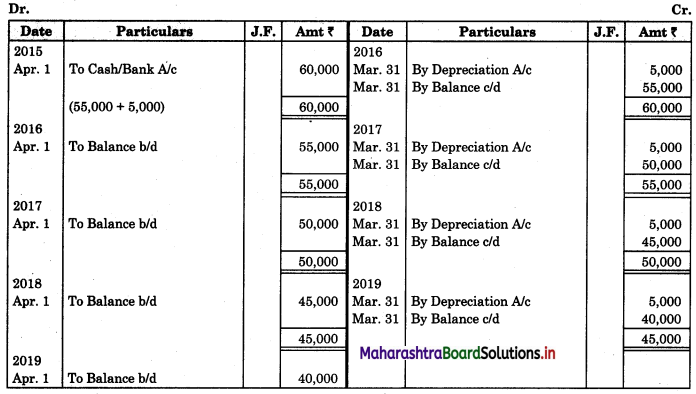Working Note:
Calculation of Depreciation per annum
Depreciation = $$\frac{Original cost of an asset (-) Scrap value}{Estimated life of asset in years}$$
= $$\frac{60,000-10,000}{10}$$
= $$\frac{50,000}{10}$$
= ₹ 5,000 p.a.

Question 2.
On 1st January 2017 ‘Sai Industries, Nagpur’ purchased a Machine costing ₹ 1,65,000 and spent ₹ 15,000 for its installation charges. The estimated life of the Machine is to be 10 years and the scrap value at the end of its life would be ₹ 30,000. On 1st October 2018, the entire Machine was sold for ₹ 1,50,000.
Show Machinery Account, Depreciation Account, for the years 2016-17, 2017-18, and 2018-19 assuming that the accounts are closed on 31st March every year.
Solution:
In the books of Sai Industries, Nagpur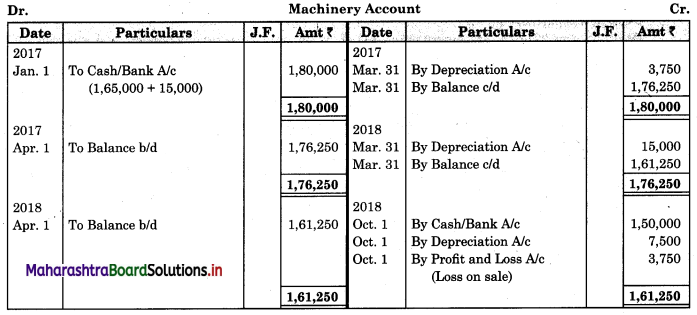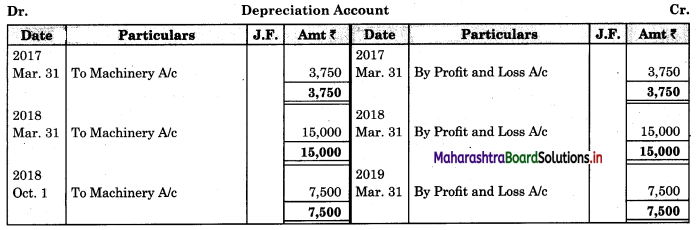Working Notes:
1. Calculation of Depreciation per annum:
Depreciation = $$\frac{Original cost of an asset (-) Scrap value}{Estimated life of asset in years}$$
= $$\frac{1,80,000-30,000}{10}$$
= $$\frac{1,50,000}{10}$$
= ₹ 15,000 p.a.2. Calculation of Profit or loss on sale of machine:
Original cost 01.01.2017 = ₹ 1,80,000
Less: Depreciation for 2016-17 (3 months) = ₹ 3,750
W.D.V. on 01-04-2017 = ₹ 1,76,250
Less: Depreciation for 2019-18 (12 months) = ₹ 15,000
W.D.V. on 01.04.2018 = ₹ 1,61,250
Less: Depreciation for 2018-19 (6 months) = ₹ 7,500
W.D.V. on date of sale = ₹ 1,53,750
Less: Selling price = ₹ 1,50,000
∴ Loss on sale of machine = ₹ 3,750

Question 3.
Shubhangi Trading Company of Dombivli purchased Machinery for ₹ 86,000 on 1st January 2016 and immediately spent ₹ 4,000 on its fixation and erection. On 1st October 2016 additional Machinery costing ₹ 40,000 was purchased.
On 1st October 2017, the Machinery purchased on 1st January 2016 became obsolete and was sold for ₹ 70,000. On 1st July 2017, a new Machine was also purchased for ₹ 45,000.
Depreciation was provided annually on 31st March at the rate of 12% per annum on the fixed installment method.
Prepare Machinery Account for three years and pass Journal Entries for the Third year i.e. 2017-2018.
Solution:
In the books of Shubhangi Trading company, Dombivli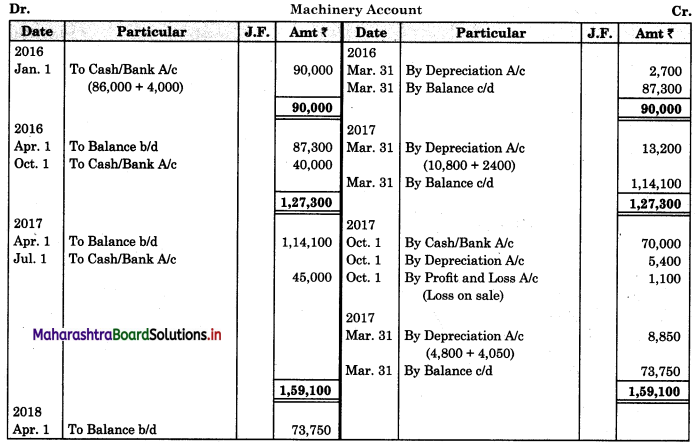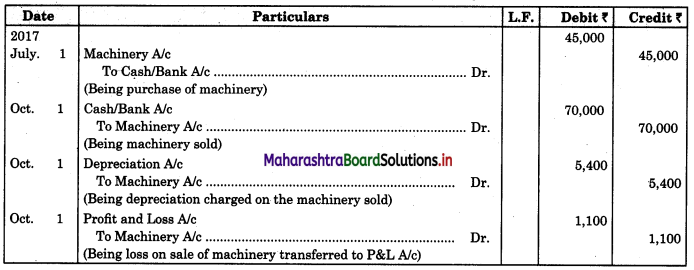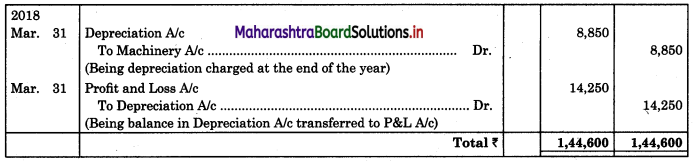Working Note:
Calculation of Profit or loss on sale of machine:
Original cost on 01.01.2016 = ₹ 90,000
Less: Depreciation for 2015-16 (3 months) = ₹ 2,700
W.D.V. on 01-04-2016 = ₹ 87,300
Less: Depreciation for 2016-17 (12 months) = ₹ 10,800
W.D.V. on 01.04.2017 = ₹ 76,500
Less: Depreciation for 2017-18 (6 months) = ₹ 5,400
W.D.V. on date of sale = 71,100
Less: Selling price = 70,000
∴ Loss on sale of machine = ₹ 1,100

Question 4.
On 1st Jan 2015, Triveni Traders Raigad purchased a Plaint for ₹ 12,000, and installation charges being ₹ 3,000. On 1st July 2016 another Plant was purchased for ₹ 25,000, on 1st April 2017 another Plant was purchased for ₹ 27,000, wages paid for installation amounted to ₹ 2,000. Carriage paid for the Plant amounted to ₹ 1,000.
Show Plant Account up to 31st March 2018 assuming that the rate of depreciation is @ 10% p.a. on Straight Line Method.
Solution: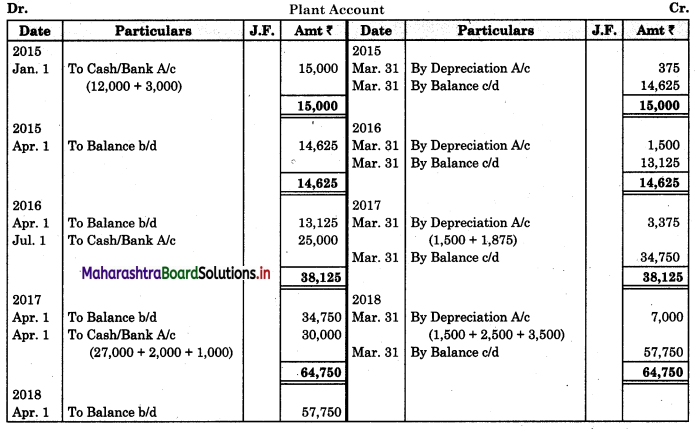Question 5.
Sameer & Company, Mumbai purchased a Machine worth ₹ 2,00,000 on 1st April 2016. On 1st July 2017, the company purchased an additional Machine for ₹ 40,000.
On 31st March 2019, the company sold the Machine purchased on 1st July 2017 for ₹ 35,000. The company writes off depreciation at the rate of 10% on the original cost and the books of accounts are closed every year on 31st March.
Show the Machinery Account and Depreciation Account for the first three years ending 31st March 2016-17, 2017-18 and 2018-19
Solution:
In the books of Sameer & Company, Mumbai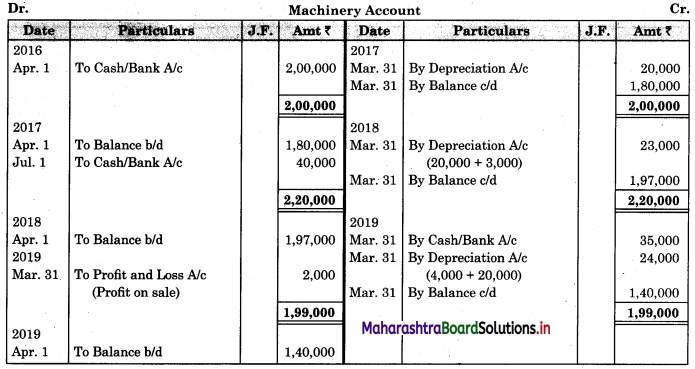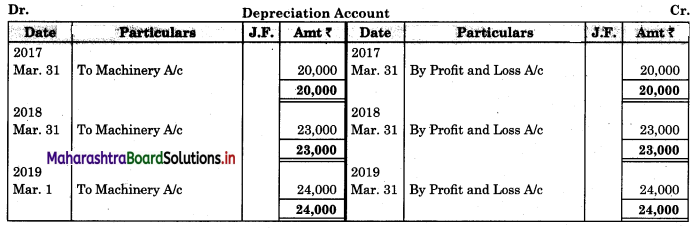Working note:
Calculation of Profit or Loss on sale of machine:
Original cost on 01.07.2017 = ₹ 40,000
Less: Depreciation for 2017-18 (9 months) = ₹ 3,000
W.D.V. on 01-04-2018 = ₹ 37,000
Less: Depreciation for 2018-19 (12. months) = ₹ 4,000
W.D.V. on date of sale = ₹ 33,000
Less: Selling price = ₹ 35,000
∴ Profit on sale of machine = ₹ 2,000

Question 6.
Samarth Manufacturing Co. Ltd, Aurangabad, purchased a New Machinery for ₹ 45,000 on 1st Jan 2015 and immediately spent ₹ 5,000 on its fixation and erection. In the same year, 1st July additional Machinery costing ₹ 25,000 was purchased. On 1st July 2016, the Machinery purchased on 1st Jan 2015 became obsolete and was sold for ₹ 40,000.
Depreciation was provided annually on 31st March at the rate of 10% per annum on the Fixed Instalment Method.
You are required to prepare Machinery Account for the year 2014-15, 2015-16, 2016-17.
Solution:
In the books of Samarth Manufacturing Co. Ltd, Aurangabad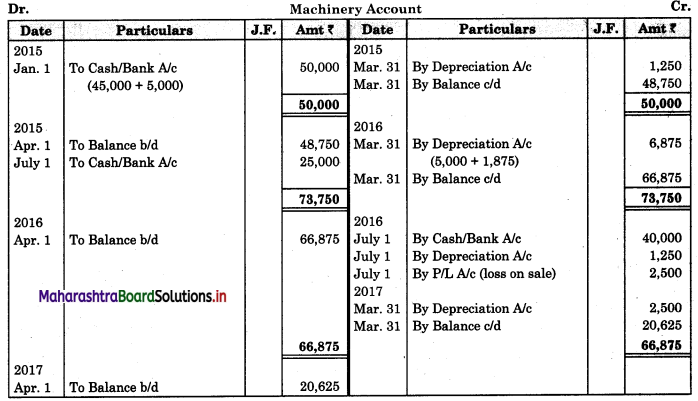Working Note:
Calculation of Profit or Loss on sale of machine:
Original cost on 01.01.2015 = ₹ 50,000
Less: Depreciation for 2014-15 (3 months) = ₹ 1,250
W.D.V. on 01-04-2015 = ₹ 48,750
Less: Depreciation for 2015-16 (12 months) = ₹ 5,000
W.D.V. on 01-04-2016 = ₹ 43,750
Less: Depreciation for 2016-17 (3 months) = ₹ 1,250
W.D.V. on date of sale = ₹ 42,500
Less: Selling price = ₹ 40,000
∴ Loss on sale of machine = ₹ 2,500

Practical Problems on Written Down Value Method

Question 1.
M/s Omkar Enterprise Jalgaon acquired a Printing Machine for ₹ 75,000 on 1st Oct 2015 and spent ₹ 5,000 on its transport and installation. Another Machine for ₹ 45,000 was purchased on 1st Jan 2017. Depreciation is charged at the rate of 20% on Written Down Value Method, on 31st March every year.
Prepare Printing Machine Account for the first four years.
Solution:
In the books of M/s Omkar Enterprise Jalgaon.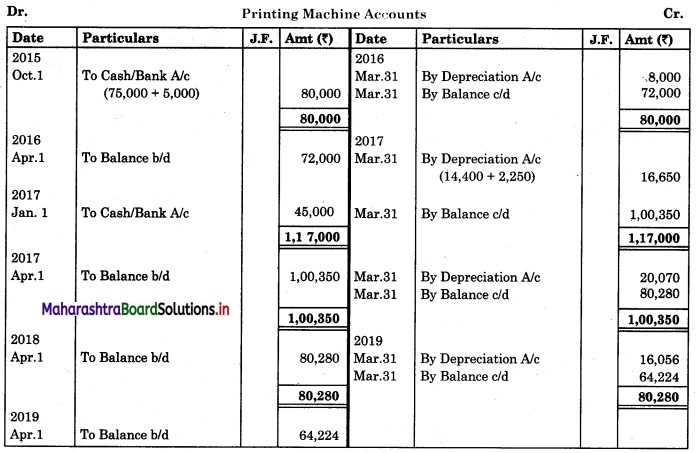Question 2.
Vishal Company, Dhule, purchased Machinery costing ₹ 60,000 on 1st April 2016. They purchased further Machinery on 1st October 2017, costing ₹ 30,000, and on 1st July 2018, costing ₹ 20,000. On 1st Jan 2019, one-third of the Machinery, which was purchased on 1st April 2016, became obsolete and it was sold for ₹ 18,000.
Assume that, company account closes on 31st March every year.
Show Machinery Account for the first three(3) years and pass journal entries for the Third year, after charging depreciation at 10% p.a. on Written Down Value Method.
Solution:
In the books of Vishal Company, Dhule.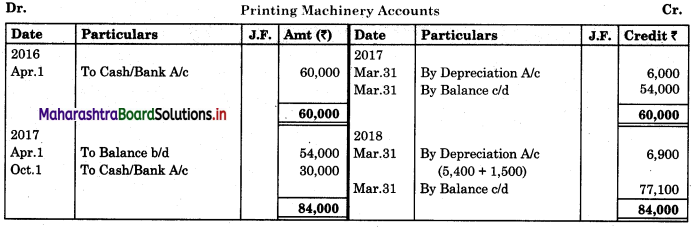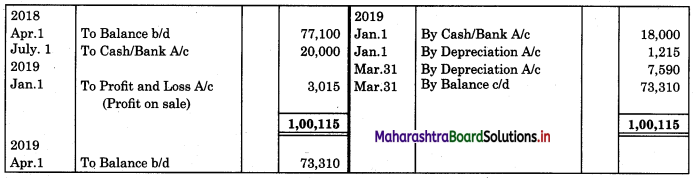Journal of Vishal Company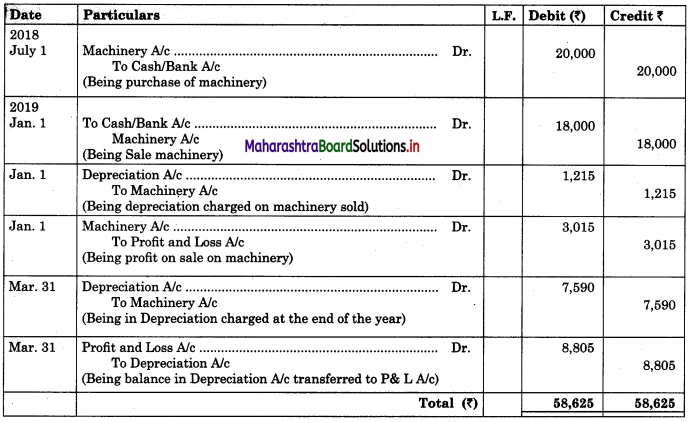Working Notes:
1. Calculation of Profit or Loss on sale as Machine:
Original cost on 01.04.2016 = ₹ 20,000
Less: Dep. for 2016-17 (12 months) = ₹ 2,000
W.D.V. on 01.04.2017 = ₹ 18,000
Less : Dep. for 2016-17 (12 months) = ₹ 1,800
W.D.V. on 01.04.2018 = ₹ 16,200
Less : Dep. for 2018-19 (9 months) = ₹ 1,215
W.D.V. on date of sale = ₹ 14,985
Less : Selling Price = ₹ 18,000
∴ Profit on sale & machine = ₹ 3,015

2. Depreciation for 2018-19
(a) Opening balance on 01.04.2018 = ₹ 77,100
Less : W.D.V. of Machine sold on 01.04.2018 = ₹ 16,200
10% depreciation on 60,900 = ₹ 6,090
(b) Purchase of Machine on 01.07.2018 20,000 – 10% – 9 months = ₹ 6,090 + ₹ 1,500 = ₹ 7,590Question 3.
Mahesh Traders Solapur purchased Furniture on 1st April 2014 for ₹ 20,000. In the same year on 1st, Oct. additional Furniture was purchased for ₹ 10,000.
On 1st Oct. 2015, the Furniture purchased on 1st April 2014 was sold for ₹ 15,000 and on the same day, a new Furniture was purchased for ₹ 20,000.
The firm charged depreciation at 10% p.a. on the Reducing Balance Method.
Prepare Furniture Account and Depreciation Account for the year ending 31st March 2015, 2016, and 2017.
Solution:
In the books of Mahesh Traders, Solapur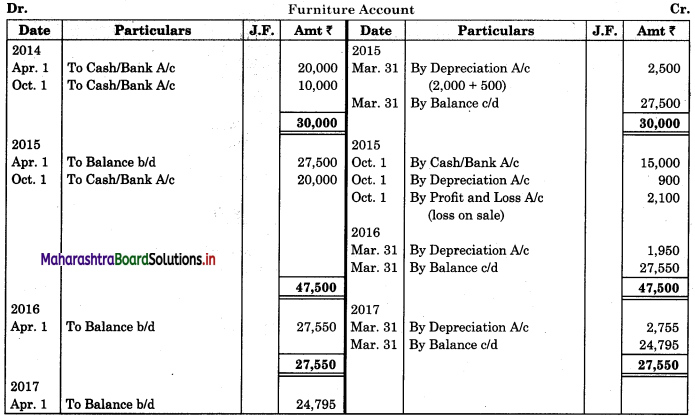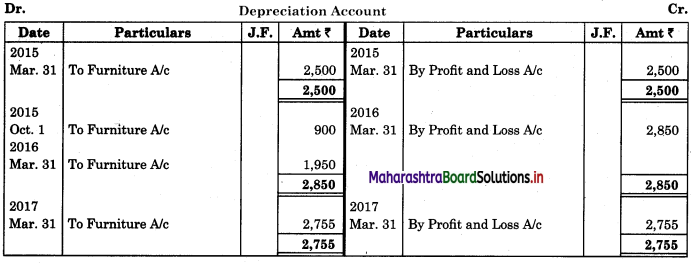Working Notes:
1. Calculation of Profit or loss on sale of furniture:
Original cost on 01.04.2014 = ₹ 20,000
Less: Depreciation for 2014-15 (12 months) = ₹ 2,000
W.D.V. on 01.04.2015 = ₹ 18,000
Less: Depreciation for 2015-16 = ₹ 900
W.D.V. on date of sale = ₹ 17,100
Less: Selling price = ₹ 15,000
∴ Loss on sale of furniture = ₹ 2,100

2. Calculation of Depreciation for 2016 -17:
(a) Opening balance on 01.04.2015 = ₹ 27,500
Less: W.D.V. of furniture sold on 01.04.2015 = ₹ 18,000
9,500 – 10% = ₹ 950
(b) Purchase of furniture on 01.10.2015 – 10% – 6months = 950 + 1,000 = ₹ 1,950

Question 4.
Radhika-Masale’ Amravati purchased a Plant on 1st Jan. 2015 for ₹ 80,000. A new Plant was also purchased
for ₹ 60,000, installation expenses being ₹ 10,000 on 1st April 2016. On 1st Jan 2017, a new Plant was purchased for ₹ 20,000, by disposing of the 1st Plant at ₹ 60,000.
Prepare Plant Account and Depreciation Account for 31st March 2015, 31st March 2016, and 31st March 2017, assuming that the rate of depreciation was @ 10% on Diminishing Balance Method.
Solution:
In the books of Radhika-Masale, Amravati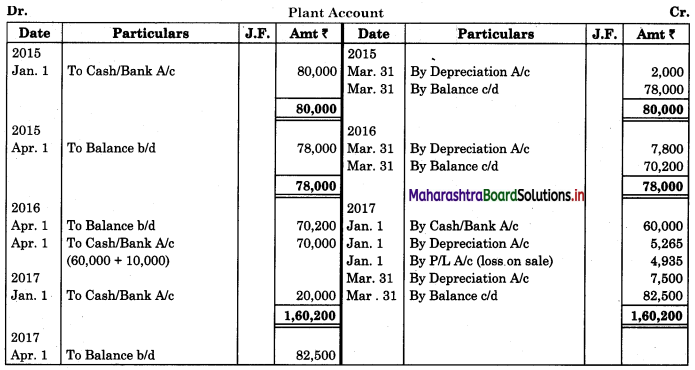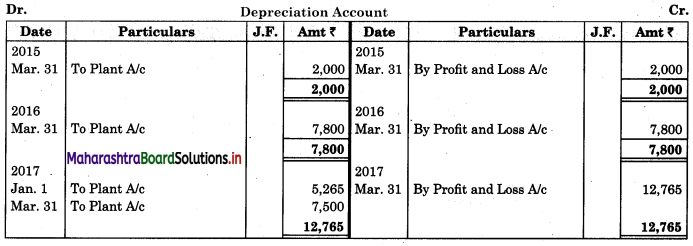Working Notes:
1. Calculation of Profit or loss on sale of plant:
Original cost on 01.01.2015 = ₹ 80,000
Less: Depreciation for 2014.15. (3 months) = ₹ 2,000
W.D.V. on 01.04.2015 = ₹ 78,000
Less: Depreciation for 2015 -16 (12 months) = ₹ 7,800
W.D.V. on 01.04.2016 = ₹ 70,200
Less: Depreciation for 2016 -17 (9 months) = ₹ 5,265
W.D.V. on date of sale = ₹ 64,935
Less: Selling price = ₹ 60,000
∴ Loss on sale of plant = ₹ 4,935

2. Calculation of Depreciation for 2016-17:
(a) Opening balance on 01.04.2016 = ₹ 70,200
Less: W.D.V. of plant sold on 01.04.2016 = ₹ 70,200
Nil – 10% = Nil
(b) Purchase of plant on 01.04.2016 – 10% – 12months = ₹ 7,000
(c) Purchase of plant on 01.01.2017 – 10% – 3m months = ₹ 500
Total = ₹ 7,500Question 5.
On 1st April 2015, Suman Traders purchased Machinery for ₹ 30,000. On 1st Oct. 2015, they purchased further Machinery costing ₹ 20,000.
On 1st Oct. 2016, they sold the Machine purchased on 1st April 2015 for ₹ 18,000 and brought another Machine for ₹ 15,000 on the same date.
Depreciation is provided on Machinery @ 20% p.a. on the Diminishing Balance Method and the financial year closes on 31st March every year.
Prepare the Machinery Account and Depreciation Account for the year 2015-16, 2016-17, and 2017-18.
Solution:
In the books of Suman Traders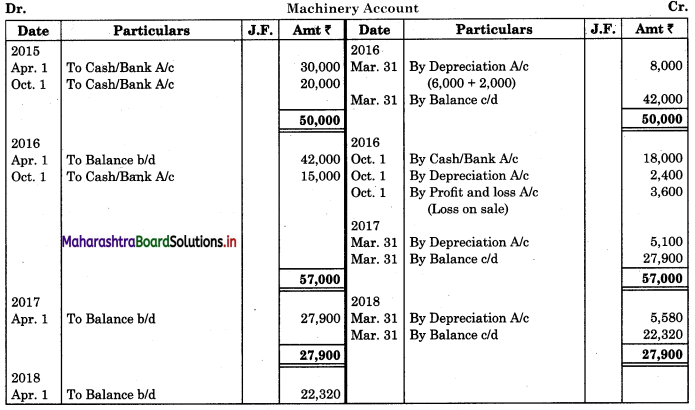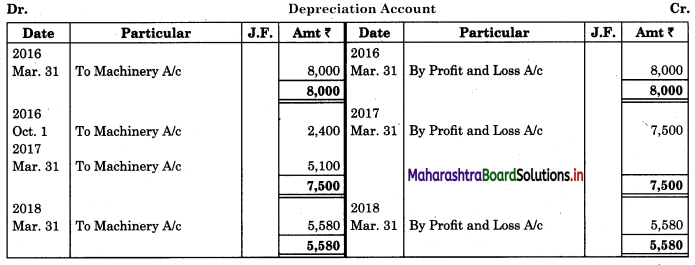Working Notes:
1. Calculation of Profit or loss on sale of machine:
Original cost on 01.04.2015 = ₹ 30,000
Less: Depreciation for 2015-16 (12 months) = ₹ 6,000
W.D.V. on 01.04.2016 = ₹ 24,000
Less: Depreciation for 2016-17 (6 months) = ₹ 2,400
W.D.V. on date of sale = ₹ 21,600
Less: Selling price = ₹ 18,000
∴ Loss on sale of machine = ₹ 3,600

2. Calculation of Depreciation for 2016-17:
(a) Opening balance on 01.04.2016 = ₹ 42,000
Less: W.D.V. of machine sold on 01.04.2016 = ₹ 24,000
18,000 – 20% = ₹ 3,600
(b) Purchase of machine on 01.10.2016 – 15,000 – 20% – 6months = 3,600 + 1,500 = ₹ 5,100